Next: Dynamic Cohesive Node Insertion Up: 1-D ANALYSIS AND RESULTS Previous: Problem Description   Contents

# Multi-Time Step Nodal Subcycling Results

Employing the multi-time step nodal subcycling algorithm presented by Smolinski (1989) and described in Chapter 2, we apply it to the simple 1-D beam problem discussed above. In order to verify our implementation of the subcycling algorithm we compare our results to those presented by Smolinski (Case) in his paper. The 1-D problem is discretized into three regions of 10 elements each, as presented in Figure 3.4. The middle region is discretized intosegments, while the other two havesegments. Using the properties selected for the reference problem, the critical time step is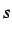, which further reduced to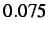to ensure that any instabilities present in the solution are a result of the algorithm and not of an inadequate time step.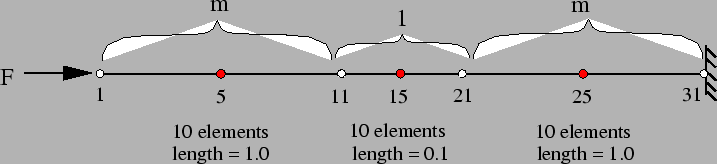As in Case, we give the two non-subcycled boundary regions a parameter of, while the middle subcycled region retains the critical time step. The simulation is run for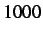time steps or, while we track the velocities of nodes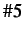,and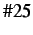, representing the middle of each of the three regions. Comparing the velocity profiles in Figures 3.5-3.7, we can see that the subcycled solution closely matches the reference solution for each node. Furthermore, the results obtained are indistinguishable from those of Casepresented by Smolinski, suggesting that our implementation is correct.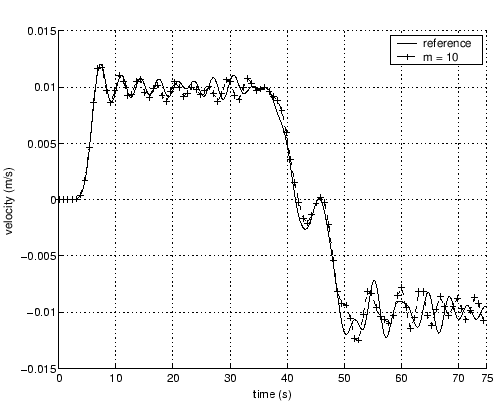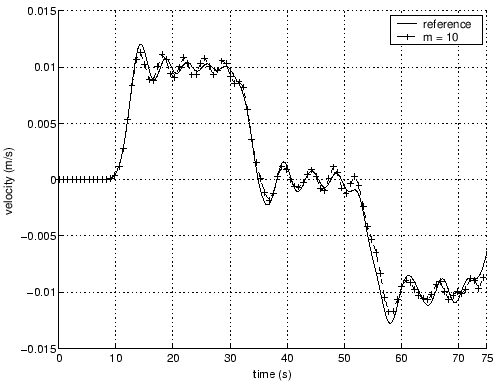Although subcycling is able to provide fairly accurate results, the level of accuracy in the non-subcycled regions decreases as their time step grows closer to the critical value for that region. The advantage of the larger time step ratio is that more computational savings is achieved since the non-subcycled regions are updated less often. Figure 3.8 shows how the increased time step ratio affects the differences in displacements and velocities of nodefor our subcycling problem at node. We can see, that as the time step ratio increases, the difference of the displacements and velocities between the reference and the subcycled cases increases. The differences at the start and end of the simulation are not displayed because the displacements differ significantly relative to each other but are quite small in respect to the rest of the simulation. As a result, they are not plotted since they would mask the effect that subcycling has on the solution. Similarly, for the velocity profile, the middle region represents a near zero velocity so that even minor differences cause large relative percentages.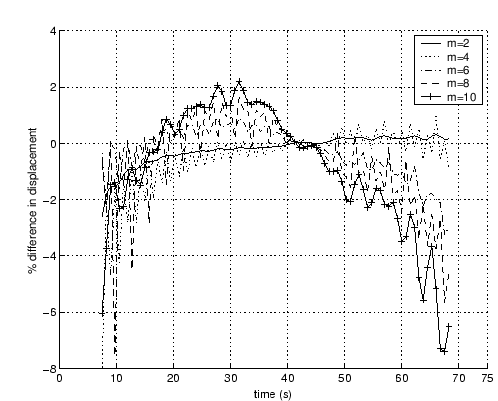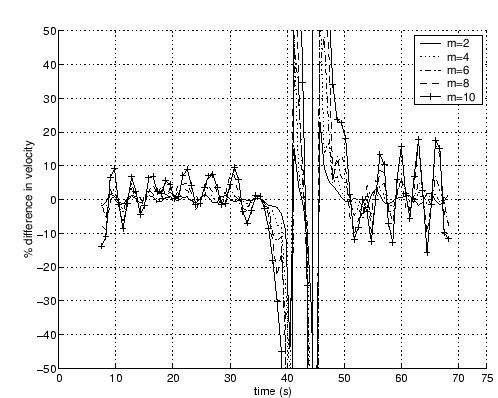In order to get some timing results and show the savings gained using the subcycling algorithm, we selected a larger problem for analysis. Figure 3.9 shows the 1-D beam which is discretized into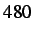equal segments each of length. The beam properties and forces are selected to match the original case presented at the beginning of this chapter. Subcycling is applied to the first and last group of elements for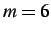,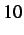and, with a time step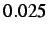(1/40th the critical time step). The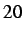inner elements are given the base time step of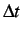, which gives us a ratio of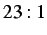, in the number of non-subcycled to subcycled nodes.The simulations are run for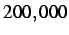time steps orfor each subcycling parameter, with the results for the internal force vector and total simulation time presented in Table 3.1. At first glance the results do not appear very favorable. Even though the time required for calculating the internal force vectors decreases, with increasing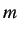, the overall time for the entire code is above the reference time. There are two reasons for this timing discrepancy. The first is that the current implementation of the subcycling algorithm may not be the most efficient. When subcycling is used the computer code must make copies of the nodal displacements, velocities and accelerations for every time step. Copying, in itself, is not very expensive but when used multiple times for each node and for each time step, this time cost can accumulate. The second reason for the timing discrepancy is that the 1-D calculation of the internal force is very simple and as a result is very fast. In higher dimensions, the complexity of the internal force vector calculation increases and has a bigger impact on the time of the solution - making it a more ideal test of the subcycling algorithm.

Table 3.1: Timing results for subcycling. The CPU time saving (in %) is given in parentheses.
 Subroutine Reference Case [s],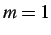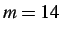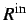10.19 6.55 ( 36%) 4.87 ( 52%) 4.45 ( 56%) Total 49.66 62.13 (-25%) 56.52 (-14%) 52.68 ( -6%)Next: Dynamic Cohesive Node Insertion Up: 1-D ANALYSIS AND RESULTS Previous: Problem Description   Contents
Mariusz Zaczek 2002-10-13